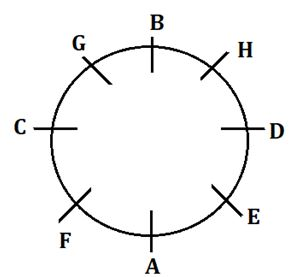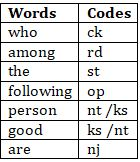Latest Banking jobs   »   Reasoning Ability Quiz For IBPS Clerk...

# Reasoning Ability Quiz For IBPS Clerk Prelims 2021- 1st August

Directions (1-5): Study the following information carefully and answer the questions given below:
Eight persons A, B, C, D, E, F, G and H are sitting on a circular table facing the centre of the table but not necessarily in the same order. H and F are facing each other. H and A are not immediate neighbours of each other. C is not immediate neighbour of H. Two persons sit between A and G. B sits immediate left of G. Two persons sit between B and E. One person sits between A and D. D and G are not immediate neighbours of each other.

Q1.Who among the following person sits immediate left of C ?
(a) G
(b) D
(c) E
(d) F
(e) None of these

Q2.How many persons sit between A and D when counted anticlockwise direction from D ?
(a) Three
(b) Four
(c) Five
(d)Two
(e) None of these

Q3.Who among the following person is facing G ?
(a) H
(b) F
(c) A
(d) E
(e) None of these

Q4.Four of the following five are alike in a certain way and hence form a group find the one which does not belong to that group ?
(a) A, D
(b) H, G
(c) G. F
(d) C, A
(e) A,E

Q5. Who among the following persons sit second to the right of H?
(a) G
(b) C
(c) B
(d) D
(e) None of these

Directions (6-10): Following questions are based on the five words given below, Study the following words and answer the following question:

MINTS RAGSE CULTS NIGAS PEMTO

Q6. If the given words are arranged in the order as they appear in a dictionary from left to right, which of the following word will be the fifth from the left end?
(a) MINTS
(b) RAGSE
(c) CULTS
(d) NIGAS
(e) PEMTO

Q7. If the letters of the given words are arranged in alphabetical order then within the word how many meaningful words will be formed?
(a) Four
(b) Three
(c) Two
(d) One
(e) None

Q8. How many letters are there between the first Letter of third word from left end and Last Letter of the second word from right end(According to the alphabetical order)?
(a) Eight
(b) Nine
(c) Ten
(d) Fifteen
(e) Sixteen

Q9. How many vowels are there in between the first letter of first word from left and last letter of last word from right (According to the alphabetical order)?
(a) Two
(b) Three
(c) None
(d) Five
(e) None of these

Q10. How many letters are there between the first letter of last word from left and first letter of the second word from left (According to the alphabetical order)?
(a) Four
(b) Fourteen
(c) Twenty
(d) Six
(e) One

Direction (11-15): Study the following information carefully and answer the questions given below
In a certain code language:
“Who among the following” is coded as “op st rd ck”
“following person who good” is coded as “nt ck ks op”
“good person following among” is coded as “rd op ks nt”
“who good person are” is coded as “nj ks nt ck”

Q11.What is the code for “following are” in the given code language?
(a) op nj
(b) nj ck
(c) op ks
(d) st rd
(e) None of these

Q12. What is the code for “are” in the given code language?
(a)ck
(b)nt
(c)ks
(d)nj
(e)None of these

Q13. What is the possible code for “person focus” in the given code language?
(a) ks tg
(b) nt tg
(c) Either (a) or( b)
(d) hc tr
(e) te we

Q14. The code “ck” is coded for which of the following word?
(a) the
(b) among
(c) following
(d) who
(e) None of these

Q15. What may be the possible code for “who based” in the given code language?
(a) op rd
(b) nt ck
(c) ck op
(d) lm rd
(e) ck lm

Solutions

Solutions (1-5):
Sol.S1. Ans. (a)
S2. Ans. (c)
S3. Ans. (d)
S4. Ans. (e)
S5. Ans. (a)

S6. Ans. (b)
Sol. Original Series: MINTS RAGSE CULTS NIGAS PEMTO
After Rearrangement: CULTS MINTS NIGAS PEMTO RAGES

S7. Ans. (e)
Sol. Original Series: MINTS RAGSE CULTS NIGAS PEMTO
After Rearrangement IMNST AEGRS CLSTU AGINS EMOPT

S8. Ans. (d)
Sol. Between (C and S) only fifteen letters are there.

S9. Ans. (c)
Sol. Between (M and O) there are No vowels.

S10. Ans. (e)
Sol. Between (P and R) there are only one letters.

Solutions (11-15):
Sol.S11. Ans. (a)
S12. Ans. (d)
S13. Ans. (c)
S14. Ans. (d)
S15. Ans. (e)#### Congratulations!Union Budget 2023-24: Free PDF